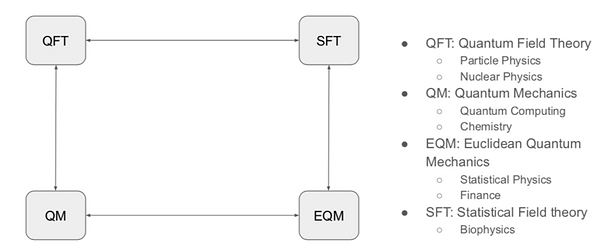Towards Derivative Pricing Thanks to Quantum Walks

by Jean Philippe Arias, Research Scientist, QuantFi

Quantum Derivative Pricing : State of the Art

QuantFi is creating quantum algorithms for finance designed to make financial analysis not only faster but also more accurate and predictive. The following references prove that it is a cutting edge topic of research:

• The referenced overview  addresses the different attempts of quantum computation to solve financial problems, as well as the expected advancements in quantum computing in finance, with improvement over classical numerical methods.

• The paper ”Quantum Mechanics and Option Pricing”  is a clear introduction to the quantum reformulation of option pricing equations. For a more complete document, we recommend B. E. Baaquie’s book .

• A Quantum Monte Carlo algorithm had already been proposed to price financial derivatives with a quadratic speed-up .

• ETH Zurich, IBM and J.P. Morgan’s research teams recently proposed an alternative methodol- ogy to price options and portfolios of options on gate-based quantum computers .

Quantum Derivative Pricing : Our Approach

• QuantFi relies on the scientific pillars of ZX-calculus, gate-based quantum computing, quantum walk- based quantum simulation, and quantum formulation of financial problems.

One of our main research topics is Quantum Derivative Pricing: we would like to handle derivatives pricing problems using quantum computing because of the quadratic or exponential quantum advantage we hope it can provide. However, quantum computing power cannot be naturally exploited in finance because of the non-quantum properties of the systems being studied.

In order to deal with this, we use B. E. Baaquie’s quantum reformulation of derivative pricing [2, 3]. This new paradigm allows us to think differently about financial phenomena by interpreting them as analogous to quantum phenomena.

In the end, this research gives us the possibility to generalize quantum computing applications to financial systems by using their properties that are analogous to quantum systems. This can be done thanks to mathematical physical tools created for more general purposes by statistical physicists, particle and nuclear physicists, and cosmologists.

Figure 1: Mathematical physical tools.

We can illustrate these mathematical physical tools thanks to figure 1. Quantum Field Theory, Quan- tum Mechanics, Euclidean Quantum Mechanics and Statistical Field Theory are four different types of physical theories with very different applications. Furthermore, they are linked to each other by mathematical operations represented by the arrows in figure 1.

An interesting point to note is that derivatives prices are modeled by processes described in the Euclidean Quantum Mechanics theory [3, 6]. In other words, we can use the mathematical link between Euclidean Quantum Mechanics and Quantum Mechanics to transform our financial systems into quantum systems. As quantum systems, their evolution can now be simulated thanks to the quantum equivalent of random walks, namely quantum walks . Simply put, we can make our derivatives systems compatible with quantum computers.

References

  R. Oru ́s, S. Mugel, and E. Lizaso, “Quantum computing for finance: Overview and prospects,” Reviews in Physics, vol. 4, p. 100028, 2019.

  B. E. Baaquie, “Quantum mechanics and option pricing,” 08 2005.

  B. E. Baaquie, “Quantum finance,” Cambridge University Press, 2007.

  P. Rebentrost, B. Gupt, and T. R. Bromley, “Quantum computational finance: Monte carlo pricing of financial derivatives,” Physical Review A, vol. 98, no. 2, p. 022321, 2018.

  N. Stamatopoulos, D. J. Egger, Y. Sun, C. Zoufal, R. Iten, N. Shen, and S. Woerner, “Option pricing using quantum computers,” arXiv:1905.02666, 2019.

  J. C. Zambrini, “Euclidean quantum mechanics,” Physical Review A, vol. 35, p. 3631–3649, Jan 1987.

  P. Arrighi, “Quantum cellular automata,” These d’habilitationa diriger les recherches, University of Grenoble, 2009.